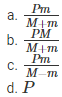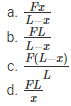# Motion of Connected Bodies MCQ for NEET

NEET  Physics is the scoring paper in the medical entrance examination. Here, you will discover the NEET Physics MCQ Questions for all Concepts as per the latest syllabus. Practice more on a regular basis with these NEET Physics objective questions on air pollution and improve your subject knowledge & problem-solving skills along with time management. NEET Physics Motion of Connected Bodies Multiple Choice Questions make you feel confident in answering the question in the exam & increases your scores to high.

## MCQ on Motion of Connected Bodies

1. A block of mass M is pulled along a horizontal frictionless surface by a rope of mass m. If P is the force exerted on the free end of the rope, what is the force exerted on the block by the rope?Answer: (b) $$\frac{P M}{M+m}$$

2. A rope of length L is pulled by a constant force F. The tension in the rope at a distance x from the end where the force is applied isAnswer: (c) $$\frac{F(L-x)}{L}$$

3. Three weights A, B and C of mass 2 kg each are hanging on string passing through a frictionless pulley. What is the tension in the string connecting weights B and C?
(a) 13 N
(b) 19.6 N
(c) 3.3 N
(d) 0

4. A frictionless pulley kept on a horizontal table connects two blocks of masses 4 kg and 5 kg. What is the acceleration of 5 kg mass?
(a) 5.44 m/s2
(b) 2.72 m/s2
(c) 19.5 m/s2
(d) 49 m/s2

5. Three blocks A, B and C each weighing 1, 8, and 27 kg respectively are connected to each other by an inextensible string and are moving on a smooth surface. If T3 is equal to 36 N, then what will T2 be equal to?
(a) 9 N
(b) 18 N
(c) 3.375 N
(d) 1.25 N

6. Three solid masses m1, m2 and m3 placed on a frictionless table and are connected in succession. If the mass m3 is dragged with a force T, the tension in the string between m2 and m3 are given asAnswer: (d) $$\frac{m_{1}+m_{2}}{m_{1}+m_{2}+m_{3}} T$$

7. A string passing over a frictionless pulley has a mass of 6 kg attached to one of its ends. To the other end a mass of 10 kg is attached. What is the tension in the thread?
(a) 2.45 N
(b) 24.5 N
(c) 73.5 N
(d) 79 N

8. What will the acceleration of the system of two masses connected to a pulley be if the masses weigh 5 kg and 10 kg?
(a) g
(b) g/2
(c) g/3
(d) g/4

9. A body of weight 2 kg is suspended as shown in the figure. What is the tension T1 in the horizontal string?
(a) $$\frac{2}{\sqrt{3}}$$
(b) $$\frac{\sqrt{3}}{2}$$
(c) 2√3
(d) 2